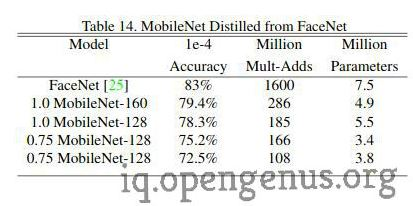MobileNet is a CNN architecture that was developed by researchers at Google in 2017 that is used to incorporate Computer Vision efficiently into small, portable devices like mobile phones and robots without significantly reducing accuracy. The total number of parameters in a standard MobileNet is 4.2 million, which is significantly lesser than some of the other CNN architectures.MobileNets also give model developers the flexibility to control the size of their model(at the cost of accuracy) depending on their requirements by introducing two new global hyperparameters that can be tuned according to the requirements of the model developer.

# Depthwise seperable convolutions

In mobilenets, depthwise seperable convolutions are used in place of standard convolutions to reduce the size and compexity of the model.

A depthwise seperable convolution is performed on a given input image in two steps:

1. Depthwise Convolution
2. Pointwise Convolution

Depthwise Convolution
In Depthwise Convolution, a seperate filter is applied to every channel of the input image individually.
Assuming that the input image is of dimension DF× DF× M(where M is the number of channels),we would require M filters of dimension DK × DK × 1 (It should be noted that each filter will be applied to only one channel of the input image). After applying a depthwise convolution on the input image, we will get M matrices of dimension (DF-DK+1) × (DF-DK+1) × 1. When these matrices are stacked together, we get a single resulting output matrix of dimension (DF-DK+1) × (DF-DK+1) × M.

Pointwise Convolution
The pointwise convolution layer applies 'N' filters of dimension 1×1×M on the output of the Depthwise Convolution layer.This results in an output image of dimension (DF-DK+1) × (DF-DK+1) × N.(The purpose of using 1×1 filters is to increase the number of channels of the output image).

Hence the total cost of computation would be DK × DK × M × (DF-DK+1) × (DF-DK+1) + M × N × (DF-DK+1) × (DF-DK+1).

A standard convolution on the other hand, filters and combines the values of the different channels of the input image in a single step.The equivalent standard convolutional layer of the depthwise seperable layer described above would contain N filters of dimension DK × DK × M(since the dimension of the input image is DF× DF× M).This would also result in an output image of dimension (DF-DK+1) × (DF-DK+1) × N.The total cost of computation will be DK × DK × M × N × (DF-DK+1) × (DF-DK+1).

To compare the two costs calculated, lets divide one with the other :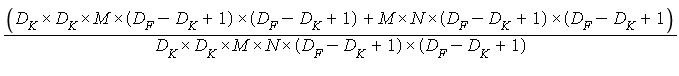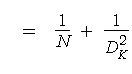Clearly the above ratio is less than 1. Hence the cost of depthwise seperable convolutions is considerably lesser than that of standard convolutions.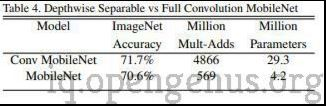It was experimentally observed that the drop in accuracy (on the ImageNet dataset) of MobileNets with depthwise seperable convolutions was only 1% when compared to a MobileNet architecture that uses standard convolutions.

# Network Structure

All of the layers use depthwise seperable convolutions layers besides the first layer.

A single mobilenet layer looks like this :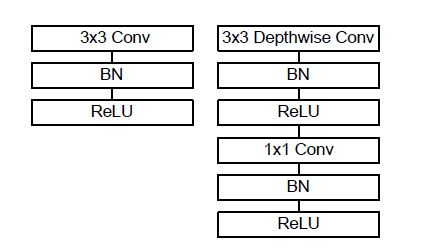The detailed architecture is given below :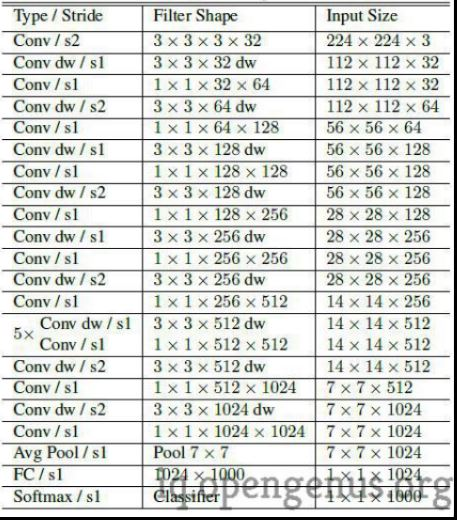Almost all of the computation is done by 1×1 convolutions that are implemented using the highly optimized general matrix multiply functions.Around 75% of the total parameters are present in 1×1 convolution layers and over 90% of the computation time is spent in these layers.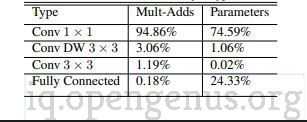Mobilenets were trained in tensorflow using RMSprop with asynchronous gradient descent. Since mobilenets are small models, they do not require regularization as they rarely overfit the data.

# Global Hyperparameters

Width Multiplier
Width muliplier (denoted by α) is a global hyperparameter that is used to construct smaller and faster(by reducing the number of computations) models.Its value lies between 0 and 1.The number of input channels(denoted by 'M') of a given layer will be reduced to αM and the number of output channels(denoted by 'N') will get reduced to αN hence reducing the cost of computation and size of the model at the cost of performance.The computation cost and number of parameters decrease roughly by a factor of α2.Some commonly used values of α are 1,0.75,0.5,0.25.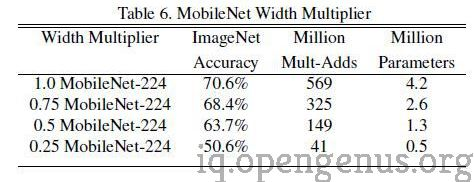Resolution Multiplier
The second parameter introduced in MobileNets is called resolution multiplier and is denoted by ρ.This hyperparameter is used to decrease the resolution of the input image and this subsequently reduces the input to every layer by the same factor. For a given value of ρ the resolution of the input image becomes 224 * ρ.This reduces the computational cost by a factor of ρ2.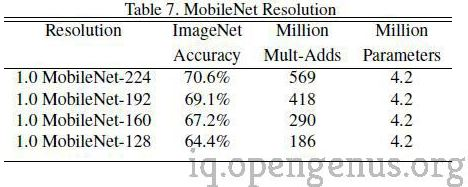The Table given below compares the performance and size of mobilenets to other popular models :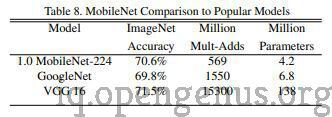# Applications of MobileNets

Fine Grained Recognition

Fine grained recognition is a field of computer vision that aims to distinguish between extremely similar object categories(like breeds of dogs).
MobileNet was trained on the Stanford Dogs Dataset(A very popular dataset that contains over 20,000 images of 120 breeds of dogs that is used for training models for Fine Grained Recognition) and achieved very impressive results.

The following table shows a comparison of the performance of some MobilNets with the Inception V3 on the Stanford Dogs Dataset :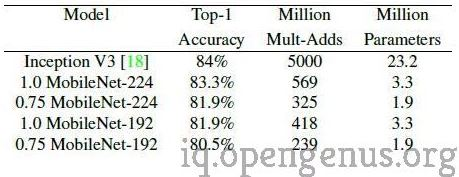Large Scale Image Geolocalization
Image geolocalization is the task of identifying where on earth a particular photograph was taken.PlaNet is a very popular Neural Network that has achieved superhuman levels of accuracy at this task.PlaNet has 52 million parameters and over 5.7 billion multiplications and additions.When the PlaNet was retrained using the MobileNet architecture, the resulting model had only 13 million parameters.Despite its small size, the accuracy of the MobileNet version of PlaNet is almost as high as the standard PlaNet.

The table given below shows a comparison of MobileNet with PlaNet and Im2GPS :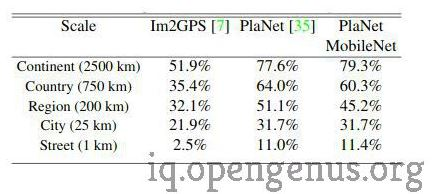Facial Attribute Classification
A face attribute classifier attempts to recognize various features of a facial image.MobileNet was used to compress a large Facial Attribute Classification model(that had 75 million parameters and 1600 million multiplication and addition operations) using knowledge distillation.
Knowledge distillation is a technique
wherein a smaller model is trained to emulate a much larger model.The resulting facial attribute classification model 3.2 million parameters and even manages to achieve better accuracy than its parent model.

The table given below summarizes the performances of the different MobileNets :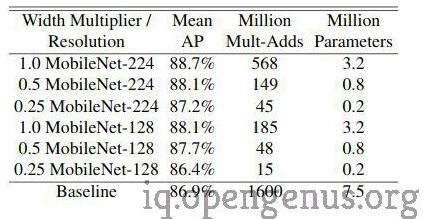Object Detection
MobileNet was trained on the COCO dataset (A very popular large scale object detection dataset) and it managed to perform almost as well as VGG and inception V2 on the 2016 COCO challenge despite its considerably small size and low computational complexity.

Face Recognition
FaceNet is a very popular face recognition model that has managed to achieve very impressive results. MobileNet was trained using knowledge distillation on FaceNet.
The table below compares the performance of mobile FaceNet with the standard FaceNet model :